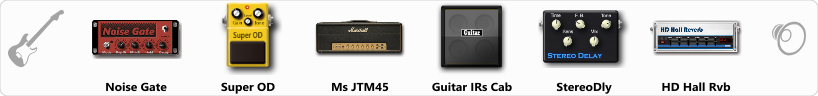Discussion in 'ToneLib-GFX presets' started by Omega Station, Aug 11, 2019.

Preset name: OmSt Post Rock Lead 1

You can find all the presets I made here: https://honestampsimreviews.com/preset-vault/ (under guitar amp sims - Tonelib GFX)

Effects chain:Effect: "Noise Gate" (Dynamics / Filter), active - "yes"
"Detect" = Gtr In
"Mode" = Manual
"Depth" = 50
"Threshold" = 60
"Attack" = 0
"Hold" = 5
"Decay" = 250

Effect: "Super OD" (Overdrive / Distortion), active - "yes"
"Drive" = 34
"Tone" = 80
"Level" = 91

Effect: "Ms JTM45" (Amp simulators), active - "yes"
"Gain" = 50
"Bass" = 47
"Middle" = 71
"Treble" = 68
"Presence" = 50
"Master" = 50
"Output" = 50
"Level (dB)" = 0

Effect: "Guitar IRs Cab" (Cabinets), active - "yes"
"Model" = Orange PPC (4x12")
"Mic Position" = Middle
"Mic Distance" = Near
"Low Cut (Hz)" = 110
"Hi Cut (kHz)" = 11.0
"Mix" = 100
"Level (dB)" = 0

Effect: "StereoDly" (Delay), active - "yes"
"Time" = Note sync, 1/8 Dotted
"Feedback" = 35
"Tone" = 76
"Sens" = 0
"Mix" = 32

Effect: "HD Hall Rvb" (Reverb), active - "yes"
"Time" = 6.7
"PreLPF" = 40
"PreDelay" = 112
"HiDamp" = 27
"LoGain" = -5.1
"Mix" = 24

Note: You will need to download and install the ToneLib-GFX software to use the preset.